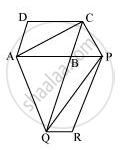# The side AB of a parallelogram ABCD is produced to any point P. A line through A and parallel to CP meets CB produced at Q and then parallelogram PBQR is completed (see the following figure). Show that ar (ABCD) = ar (PBQR). - Mathematics

The side AB of a parallelogram ABCD is produced to any point P. A line through A and parallel to CP meets CB produced at Q and then parallelogram PBQR is completed (see the following figure). Show that

ar (ABCD) = ar (PBQR).

[Hint: Join AC and PQ. Now compare area (ACQ) and area (APQ)]#### SolutionLet us join AC and PQ.

ΔACQ and ΔAQP are on the same base AQ and between the same parallels AQ and CP.

∴ Area (ΔACQ) = Area (ΔAPQ)

⇒ Area (ΔACQ) − Area (ΔABQ) = Area (ΔAPQ) − Area (ΔABQ)

⇒ Area (ΔABC) = Area (ΔQBP) ... (1)

Since AC and PQ are diagonals of parallelograms ABCD and PBQR respectively,

∴ Area (ΔABC) = 1/2Area (ABCD) ... (2)

Area (ΔQBP) = 1/2Area (PBQR) ... (3)

From equations (1), (2), and (3), we obtain

1/2Area (ABCD) = 1/2Area (PBQR)

Area (ABCD) = Area (PBQR)

Concept: Corollary: Triangles on the same base and between the same parallels are equal in area.
Is there an error in this question or solution?
Chapter 9: Areas of Parallelograms and Triangles - Exercise 9.3 [Page 163]

#### APPEARS IN

NCERT Class 9 Maths
Chapter 9 Areas of Parallelograms and Triangles
Exercise 9.3 | Q 9 | Page 163

Share